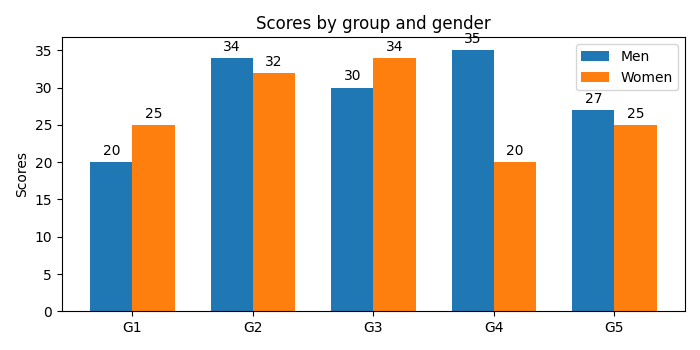# How to add group labels for bar charts in Matplotlib?

To make grouped labels for bar charts, we can take the following steps −

• Create lists for labels, men_means and women_means with different data elements.

• Return evenly spaced values within a given interval, using numpy.arrange() method.

• Set the width variable, i.e., width=0.35.

• Create fig and ax variables using subplots method, where default nrows and ncols are 1.

• The bars are positioned at *x* with the given *align*\ment. Their dimensions are given by *height* and *width*. The vertical baseline is *bottom* (default 0), so create rect1 and rect2 using plt.bar() method.

• Set the Y-Axis label using plt.ylabel() method.

• Set a title for the axes using set_title() method.

• Get or set the current tick locations and labels of the X-axis, using set_xticks() method.

• Set X-axis tick labels of the grid, using set_xticklabels() method.

• Place a legend on the figure, using legend() method.

• Annotate created bars (rect1 and rect2) with some label using autolabel() method, that is userdefined method.

• To show the figure, use the plt.show() method.

## Example

import matplotlib.pyplot as plt
import numpy as np

plt.rcParams["figure.figsize"] = [7.00, 3.50]
plt.rcParams["figure.autolayout"] = True
labels = ['G1', 'G2', 'G3', 'G4', 'G5']
men_means = [20, 34, 30, 35, 27]
women_means = [25, 32, 34, 20, 25]
x = np.arange(len(labels))
width = 0.35
fig, ax = plt.subplots()
rects1 = ax.bar(x - width / 2, men_means, width, label='Men')
rects2 = ax.bar(x + width / 2, women_means, width, label='Women')
ax.set_ylabel('Scores')
ax.set_title('Scores by group and gender')
ax.set_xticks(x)
ax.set_xticklabels(labels)
ax.legend()

def autolabel(rects):
for rect in rects:
height = rect.get_height()
ax.annotate('{}'.format(height),
xy=(rect.get_x() + rect.get_width() / 2, height),
xytext=(0, 3), # 3 points vertical offset
textcoords="offset points",
ha='center', va='bottom')

autolabel(rects1)
autolabel(rects2)
plt.show()

## Output